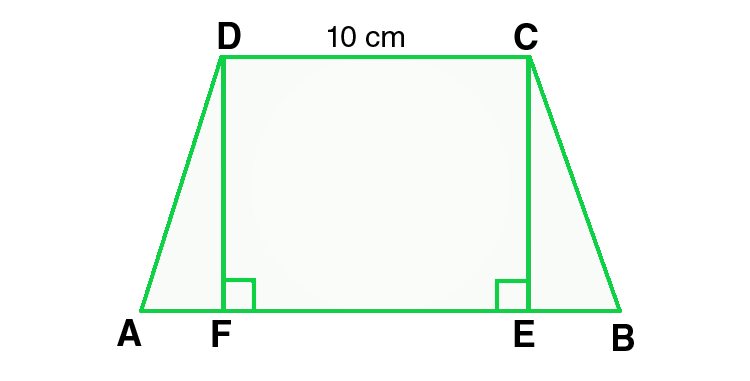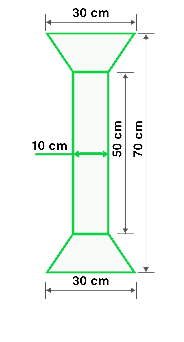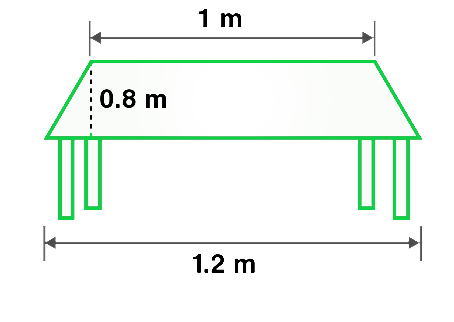# Class 8 RD Sharma Solutions – Chapter 20 Area Of Trapezium And Polygon- Exercise 20.2 | Set 1

• Last Updated : 16 Apr, 2021

### Question 1. Find the area, in square meters, of the trapezium whose bases and altitudes are as under :

(i) bases = 12 dm and 20 dm, altitude = 10 dm

(ii) bases = 28 cm and 3 dm, altitude = 25 cm

Attention reader! All those who say programming isn't for kids, just haven't met the right mentors yet. Join the  Demo Class for First Step to Coding Coursespecifically designed for students of class 8 to 12.

The students will get to learn more about the world of programming in these free classes which will definitely help them in making a wise career choice in the future.

(iii) bases = 8 m and 60 dm, altitude = 40 dm

(iv) bases = 150 cm and 30 dm, altitude = 9 dm

Solution:

Area of trapezium = 1/2 (Sum of lengths of parallel sides) × altitude

(i) Given:

Length of bases of trapezium = 12 dm and 20 dm,

Length of altitude = 10 dm

As we know that 10 dm = 1 m

Therefore, Length of bases in m is 1.2 m and 2 m.

Similarly, length of altitude in m is 1 m.

Now, put the values in the formula(i.e. Area of trapezium),

Area of trapezium = 1/2 (1.2 + 2.0) × 1 =  1/2 × 3.2 = 1.6

Hence, Area of trapezium = 1.6m2

(ii) Given:

Length of bases of trapezium = 28 cm and 3 dm,

Length of altitude = 25 cm,

As we know that 10 dm = 1 m

Therefore, Length of bases in m = 0.28 m and 0.3 m

and length of altitude in m = 0.25 m

Now, put the values in the given formula(i.e. Area of trapezium),

Area of trapezium = 1/2 (0.28 + 0.3) × 0.25 = 1/2 × 0.58× 0.25 = 0.0725

Hence, Area of trapezium = 0.0725m2

(iii) Given:

Length of bases of trapezium = 8 m and 60 dm,

Length of altitude = 40 dm.

As we know that 10 dm = 1 m

Therefore, Length of bases in m = 8 m and 6 m

and length of altitude in m = 4 m

Now, put the values in the given formula(i.e. Area of trapezium),

Area of trapezium = 1/2 (8 + 6) × 4 = 1/2 × 56 = 28

Hence, Area of trapezium = 28m2

(iv) Given:

Length of bases of trapezium = 150 cm and 30 dm,

Length of altitude = 9 dm.

As we know that 10 dm = 1 m

Therefore, Length of bases in m = 1.5 m and 3 m

and length of altitude in m = 0.9 m.

Now, put the values in the given formula(i.e. Area of trapezium),

Area of trapezium = 1/2 (1.5 + 3) × 0.9 = 1/2 × 4.5 × 0.9 = 2.025

Hence, Area of trapezium = 2.025m2

### Question 2. Find the area of a trapezium with base 15 cm and height 8 cm, if the side parallel to the given base is 9 cm long.

Solution:

Given:

Length of bases of trapezium = 15 cm and 9 cm,

Length of altitude = 8 cm.

As we know that,

Area of trapezium = 1/2 (Sum of lengths of parallel sides) × altitude

Now, put all the given values in this formula, and we get,

Area of trapezium = 1/2 (15 + 9) × 8 = 1/2 × 192 = 96

Hence, the area of trapezium = 96m2

### Question 3. Find the area of a trapezium whose parallel sides are of length 16 dm and 22 dm and whose height is 12 dm.

Solution:

Given:

Length of bases of trapezium = 16 dm and 22 dm,

Length of altitude = 12 dm.

As we know that 10 dm = 1 m

Therefore, the length of bases in m = 1.6 m and 2.2 m

and length of altitude in m = 1.2 m.

As we know that,

Area of trapezium = 1/2 (Sum of lengths of parallel sides) × altitude

Now, put all the given values in this formula, and we get,

Area of trapezium = 1/2 (1.6 + 2.2) × 1.2 = 1/2 × 3.8 × 1.2 = 2.28

Hence, the Area of trapezium = 2.28m2

### Question 4. Find the height of a trapezium, the sum of the lengths of whose bases (parallel sides) is 60 cm and whose area is 600 cm2.

Solution:

Given:

Length of bases of trapezium = 60 cm,

Area = 600 cm2

As we know that,

Area of trapezium = 1/2 (Sum of lengths of parallel sides) × altitude

Now, put all the given values in this formula, and we get,

600 = 1/2 (60) × altitude

600 = 30 × altitude

altitude = 600/30 = 20

Hence, the length of altitude is 20 cm.

### Question 5. Find the altitude of a trapezium whose area is 65 cm2 and whose base is 13 cm and 26 cm.

Solution:

Given:

Length of bases of trapezium = 13 cm and 26 cm,

Area = 65 cm2

As we know that,

Area of trapezium = 1/2 (Sum of lengths of parallel sides) × altitude

Now, put all the given values in this formula, and we get,

65 = 1/2 (13 + 26) × altitude

65 = 39/2 × altitude

altitude = (65×2) /39 = 130/39 = 10/3

Hence, the length of altitude = 10/3 cm

### Question 6. Find the sum of the lengths of the bases of a trapezium whose area is 4.2 m2 and whose height is 280 cm.

Solution:

Given:

Height of trapezium = 280 cm = 2.8m,

Area = 4.2 m2,

As we know that,

Area of trapezium = 1/2 (Sum of lengths of parallel sides) × altitude

We can also rewrite the formula as,

Sum of lengths of parallel sides = (2 × Area) / altitude

= (2 × 4.2) / 2.8 = 8.4/2.8 = 3

Hence, the sum of lengths of parallel sides = 3 m

### Question 7. Find the area of a trapezium whose parallel sides of lengths 10 cm and 15 cm are at a distance of 6 cm from each other. Calculate this area as,

(i) The sum of the areas of two triangles and one rectangle.

(ii) The difference of the area of a rectangle and the sum of the areas of two triangles.

Solution:(i) As we know that,

Area of a trapezium ABCD = area of ∆DFA + area of rectangle(DFEC) + area of ∆CEB

= (1/2 × AF × DF) + (FE × DF) + (1/2 × EB × CE)

= (1/2 × AF × h) + (FE × h) + (1/2 × EB × h)

= 1/2 × h × (AF + 2FE + EB) = 1/2 × h × (AF + FE + EB + FE)

= 1/2 × h × (AB + FE)

= 1/2 × h × (AB + CD)           -(Opposite sides of rectangle are equal)

= 1/2 × 6 × (15 + 10) = 1/2 × 6 × 25 = 75

Hence, the area of trapezium = 75 cm2

(ii) Area of rectangle ABEF = 15 x 6 = 90 cm2

Area of ∆ADF + ∆BEC = 1/2 (FD + CE) x DM

= 1/2 x (15 – 10) x 6 = 1/2 x 5 x 6 = 15 cm2

Area of trapezium = 90 – 15 = 75 cm²           -(Area of rectangle – Area of two triangles)

Hence the difference of the area of a rectangle and

the sum of the areas of two triangles is 75 cm2

### Question 8. The area of a trapezium is 960 cm2. If the parallel sides are 34 cm and 46 cm, find the distance between them.

Solution:

As we know that,

Area of trapezium = 1/2 (Sum of lengths of parallel sides) × distance between parallel sides(height)

Also, we can rewrite the formula as,

Distance between parallel sides = (2 × Area) / Sum of sides

Now, put all the given values in this formula, and we get,

= (2 × 960) / (34 + 46) = (2 × 960) / 80 = 1920/80 = 24

Hence, the distance between parallel sides = 24 cm

### Question 9. Find the area of Fig. as the sum of the areas of two trapeziums and a rectangle.Solution:

Given:

Length of rectangle = 50 cm

Breadth of rectangle = 10 cm

Length of the parallel sides of the trapezium = 30 cm and 10 cm

Distance between parallel sides of trapezium = (70 – 50)/2 = 20/2 = 10

So, the height of the trapezium is 10cm

From the given figure we conclude that,

Area of figure = Area of two trapeziums + Area of rectangle

= 2 × 1/2 (Sum of lengths of parallel sides) × altitude + Length × Breadth

Now, put all the given values in this formula, and we get,

= 2 × 1/2 (30+10) × 10 + 50 × 10 = 40 × 10 + 50 × 10

= 400 + 500 = 900

Hence, the area of figure = 900 cm2

### Question 10. Top surface of a table is trapezium in shape. Find its area if its parallel sides are 1 m and 1.2 m and the perpendicular distance between them is 0.8 m.Solution:

Given:

Length of the parallel sides of the trapezium = 1.2m and 1m,

Distance between parallel sides of trapezium(height) = 0.8m

As we know that,

Area of trapezium = 1/2 (Sum of lengths of parallel sides) × distance between parallel sides

Now, put all the given values in this formula, and we get,

Area of trapezium = 1/2 (1.2 + 1) × 0.8 = 1/2 × 2.2 × 0.8 = 0.88

Hence, the area of trapezium = 0.88m2

### Chapter 20 Area Of Trapezium And Polygon- Exercise 20.2 | Set 2

My Personal Notes arrow_drop_up